# Assignment 6 Information

• Support code:

• a6code.com --- support code Version 1.2
• a6data.scm --- data sets
• assign6.scm --- a file with "stubs" for your procedures

• There is a variable print-info whose default value is #t. If you want to turn off all printing in the support code, set it to #f.

• Also, the procedure test-dtree described in the handout was actually called test in the support code. However, I've defined test-dtree in the latest version of the support code.

• There are procedures print printifin that take a variable number of arguments which will are display'ed to the screen. The printif procedure does not print anything if the print-info variable is #f.

• Information on problems:

• Electronic submission information

whuang@cs.rpi.edu; email

## Problem 3: Chi-squared pruning

The purpose of chi-squared pruning is to test whether splitting on an attribute contributes a statistically significant amount of information. This is covered in our text on pages 542--3, but you may find the following explanation clearer.

NOTE: The converter I used to create images from the mathematical equations and symbols wasn't working exactly right; that's why you see the horizontal and vertical bars below.

NOTE: In the following discussion, the two classifications "positive" and "negative" are used. For Problem 3 you may assume that there are just two classifications, but you should NOT make any assumptions as to exactly what they are (e.g. yes, no, positive, negative, edible, poisonous, etc.) It's probably not that much more difficult to write your code so that it works with an arbitrary number of classifications.

The chi-squared test is a measurement of whether splitting on a given attribute matters. Before splitting on an attribute, we can characterize how many examples there are for each classification and see how closely each split follows these proportions. If the attribute doesn't matter, then we'd expect that each "split" of training data (as divided by this attribute) to have approximately the same fraction of examples in each classification as the training data did before splitting on this attribute

Suppose the current call to the learn-dtree is given a set of training data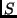. These data have mixed classifications; the number of positive examples inis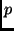, and the number of negative examples is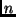. We know that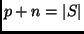.

Through the information analysis, we have picked an attribute that splitsinto a number of subsets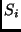. Each of these subsets will (in general) have mixed classifications. Let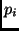be the number of positive examples inand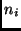be the number of negative examples. We know that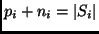.

If this attribute was irrelevant, then we would expect that the number of positive examples inwould be: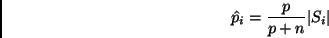Likewise, we would expect the number of negative examples to be: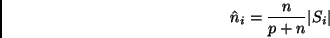Now, we calculate how much error'' there is between the actual number of positive and negative examples in eachand the expected number.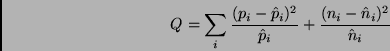Note that this sum is over each subset created by the split on this attribute. If there are three values for this attribute, then i goes from 1 to 3 in the sum above.

If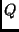is small, that means that the attribute is not relevant -- the data in each split are following the same distribution as the data before splitting on this attribute. Ifis large, that means that there is a lot of "error" between what we would have expected (under the irrelevant attribute hypothesis) and the actual distribution of examples.

Thedistribution will determine the probability that the attribute is not relevant. I have provided a Scheme implementation of this function in the support code:

  (chi^2 dof Q)

You should use
dof = (number of values of this attribute) - 1


The chi^2 procedure will return a probability between 0 and 1 which indicates that our hypothesis (that this attribute doesn't matter) is correct. We will set a low threshold for this test: if the probability is greater than 0.001, then we will assume that the hypothesis is correct.

Although this threshold may seem low, consider the following quote from "Numerical Recipes in C"

It is not uncommon to deem acceptable on equal terms any models with, say, Q > 0.001. This is not as sloppy as it sounds: truly wrong models will often be rejected with vastly smaller values of Q, 1e-18, say.

If the "irrelevance hypothesis" is deemed correct, then you should not split on this attribute -- instead just pick the majority classification.

For your information, my Scheme implementation of the chi^2 function follows the algorithm from the book "Numerical Recipes in C" for computing the gammq function.

## Problem 4: testing and comparison

For this (written) problem, turn in the following:

1. Plot a learning curve (as described below) using your learn-dtree procedure.

2. Run your learn-dtree and chi^2-learn-dtree procedures on the loan-data and the mushroom-data1. Show the resulting decision trees and explain the difference(s) between the trees learned with and without the chi-squared pruning. You should go into some detail, but your answer should not exceed 2 pages. You should be able to answer this part of Problem 4 in 1 page, though.

### Learning curves

In order to plot learning curves, you should repeat the following for actual training data set sizes of 5, 10, 15, 20, 50, 80, 110, 140, 170, and 200.

• Select the actual training data randomly from the mushroom-data1. I have provided a procedure in the support code to do this for you:
(pick-random-subset s size)

This will randomly pick "size" elements from the list "s".

• Train a decision tree on these training data using your learn-dtree procedure

• Test this decision tree on those same training data (using the test procedure from the support code).

• Test this decision tree on the mushroom-data2 (using the test procedure again).

You should find it simple to write a procedure to help you run these tests. Show the performance on the training data and the testing data on the same plot. (The x axis should be the data set size, and the y axis should be the fraction of examples classified correctly. There should be two curves on the plot: one for performance on the training data and one for the testing data.)

## (Bonus) Problem 5: discretize

For this problem:
• Write the procedure:
(discretize-attribute training-data attribute-names attribute)

which will discretize a single attribute specified by the "attribute" argument. It should return a function that will discretize the specified attribute on a data set. Here's an example discretize-attribute function that picks a random threshold for the given attribute:
(define (discretize-attribute training-data attribute-names attribute)
(let* ((attribute-pos (- (length attribute-names)
(length (member attribute attribute-names))))
(threshold (+ (random 10) 18)))
(display "The randomly picked threshold is ")
(display threshold)
(newline)
(lambda (data-set)
(map (lambda (example)
(let ((class (car example))
(attributes (cadr example)))
(list class
(append (list-head attributes attribute-pos)
(if (> (list-ref attributes attribute-pos)
threshold)
'(high)
'(low))
(list-tail attributes (+ attribute-pos 1))))))
data-set))))

Here's how it works on the following data set to discretize the "age" attribute:
(define test-data '((healthy (22 ok  low))
(ill     (23 bad medium))
(ill     (19 bad high))
(healthy (25 bad low))
(ill     (14 ok medium))
(ill     (23 ok medium))
(healthy (35 bad low))))

(define test-names '(age blood-pressure height))

(define disc-fn (distretize-attribute test-data test-names 'age))
The randomly picked threshold is 22

(disc-fn test-data)
; Value: ((healthy (low ok low))
(ill     (high bad medium))
(ill     (low bad high))
(healthy (high bad low))
(ill     (low ok medium))
(ill     (high ok medium))
(healthy (high bad low)))

Although here I've used a binary attribute, you code could use an attribute with more than two values. Note that you will have to use "generic" attribute values (e.g. "low" and "high" since there is no provision to supply a list of attribute values that might make sense with the given attribute.)

• Test your procedure and evaluate its performance using the subset of the "Wisconsin Breast Cancer database" in the file bc-data.scm. You should:

• create discretize functions from a training data set
• apply them to the training data to create a discrete data set
• learn a decision tree from these discretized training data
• apply the same discretize functions to a test data set
• test your decision tree against the distretized test data

The examples in this database consist of a number of continuous-valued measurements of a tissue sample; the classification is whether the sample is malignant or benign. Since this database consists entirely of continuous attributes, you can either discretize all of them, or you can use a subset of them, i.e. just remove the attributes that you haven't discretized.

The measurements were done automatically from digitized images. You can see some of the original images at http://dollar.biz.uiowa.edu/~street/xcyt/images/.

You should turn in your code for this problem through the Assignment 5 web tester. This web tester will not run any tests but will simply collect your code. You should turn in on paper a short (definitely no more than 2 or 3 pages) description and analysis of how your discretize function did on the Wisconsin breast cancer database.